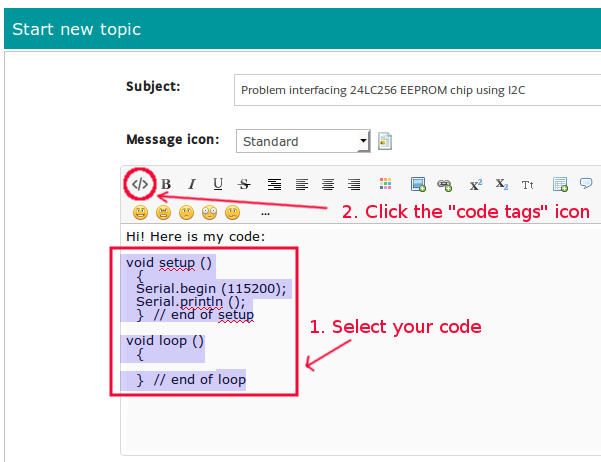# help

i use arduino with water flow sensor , i want to know an idea to know if the flow rate is constant for a period of time if yes turn on a led >> thanks and sorry for bad english

OK, so you have an Arduino and you have a flow sensor and you have a sketch that is working.

i have a variable that holds value which changes every one second >> i want to know if this value be constant for a period of time , if yes > turn on a led

I think requiring the flow to be constant might be a problem, but if it's sufficient for it to be within some limits that you will set then everything else seems feasible.

The way I'd approach this is to have a variable holding the value of millis() last time the flow was seen to be outside the required limits. Use the technique demonstrated in the blink without delay example sketch to find how long ago that value was updated and hence whether the flow condition has been met for long enough to turn the LED on.

I guess you would also want to turn the LED off as soon as the flow was seen to be outside the required limits.

This is not very specific, so the help you will get is not specific.

The 'period of time' will need to be defined somehow. If you are changing the value every second, when you change the value you should check if it has changed. If it has not, increment a variable. Once the variable hits a specific value you can turn on the LED.

At some point you will need to turn the LED off. Have you thought about that?

How to use this forum

• Moderator

sorry for disturbance ... " a variable holding the value of millis() last time the flow was seen to be outside the required limits. " can you illustrate please

volatile int NbTopsFan;
int Calc;
int hallsensor = 3;
int led = 13 ;

void rpm ()
{
NbTopsFan++;

}

void setup()
{
pinMode(hallsensor,INPUT );
pinMode(led,OUTPUT );
Serial.begin(9600);

}

void loop ()

{
NbTopsFan = 0;// its counter of no of puleses in 1 second.
attachInterrupt(1, rpm, RISING);
delay (1000);
detachInterrupt(1);
Calc = (NbTopsFan * 60 / 7.5); // flow rate in leter per minute .
}

this is the code and i need that if the variable Calc is within a specific range for a period of time (for example 1 minute) , a led is turned on , else led stay at off state >>

Use if (Calc > lower && Calc < higher) to detect you are in the range you want.
Start a watch.
If you are in range for one minute turn on the LED.
If a minute goes by and you are not in the range turn off the LED

Edit:
We assume the water flow sensor is not a simple contact, if so you will have to worry about bouncing.

but i do not know the lower and higher values , what i want that if the Calc variable is approximately constant for a 1 minute > turn on a led , sorry for disturbance

Read this before posting a programming questioni do not know the lower and higher values

You have to decide what these should be.

what i want that if the Calc variable is approximately constant

Lets say "approximately constant" is 200 then YOU have to come up with a reasonable "lower" value, lets say it will be 195 and a reasonable "higher" value, let's say it will be 205.

So you would have: (Calc > 195 && Calc < 205)

i do not have the value of approximately constant .. when the value become constant regardless of its value >> the led is turned on .

Take 10 or 100 or 1000 etc. readings if they are equal to each other turn on the LED.

thank you very much >> it works well now >> and thanks for all for your care